Скачать презентацию FIXED-INCOME SECURITIES Chapter 2 Bond Prices and Yields

• Количество слайдов: 27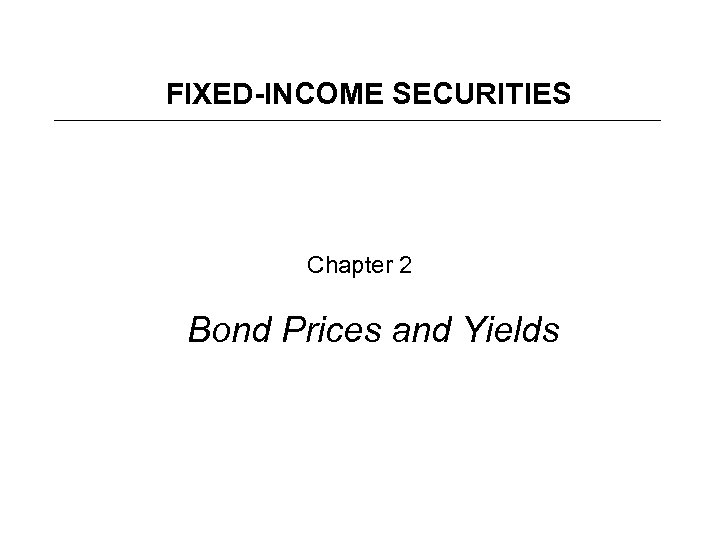FIXED-INCOME SECURITIES Chapter 2 Bond Prices and Yields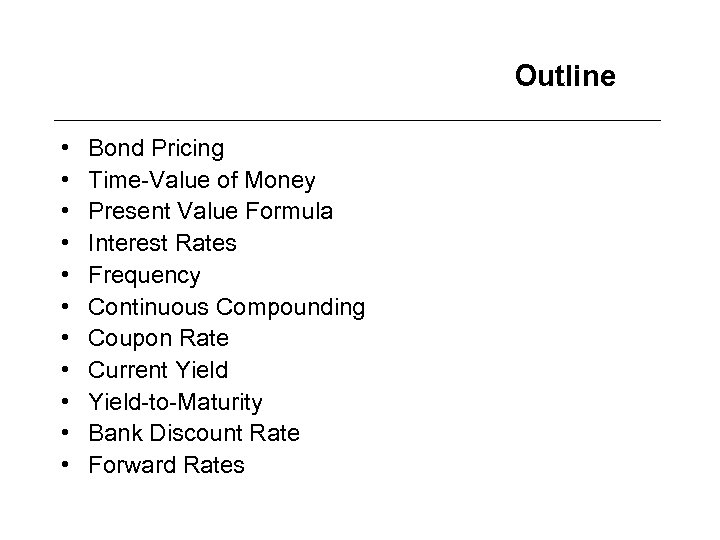Outline • • • Bond Pricing Time-Value of Money Present Value Formula Interest Rates Frequency Continuous Compounding Coupon Rate Current Yield-to-Maturity Bank Discount Rate Forward Rates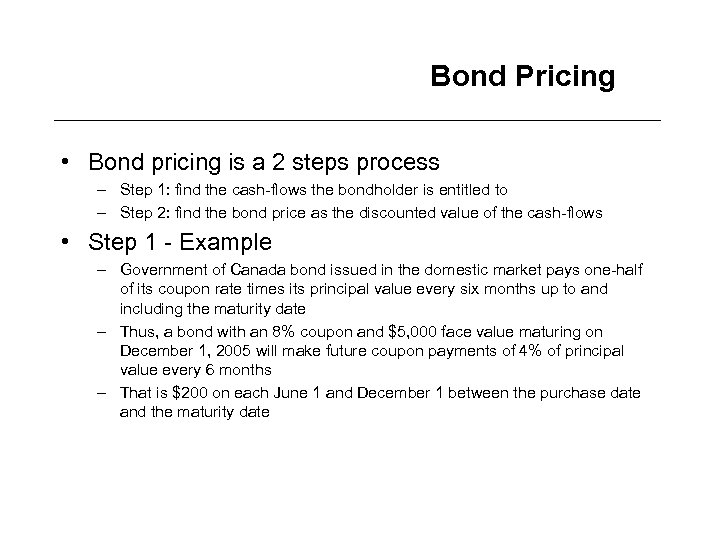Bond Pricing • Bond pricing is a 2 steps process – Step 1: find the cash-flows the bondholder is entitled to – Step 2: find the bond price as the discounted value of the cash-flows • Step 1 - Example – Government of Canada bond issued in the domestic market pays one-half of its coupon rate times its principal value every six months up to and including the maturity date – Thus, a bond with an 8% coupon and \$5, 000 face value maturing on December 1, 2005 will make future coupon payments of 4% of principal value every 6 months – That is \$200 on each June 1 and December 1 between the purchase date and the maturity date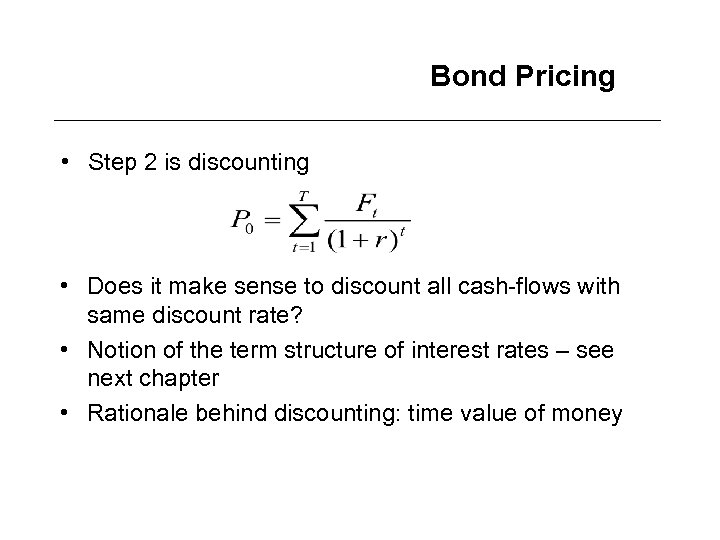Bond Pricing • Step 2 is discounting • Does it make sense to discount all cash-flows with same discount rate? • Notion of the term structure of interest rates – see next chapter • Rationale behind discounting: time value of money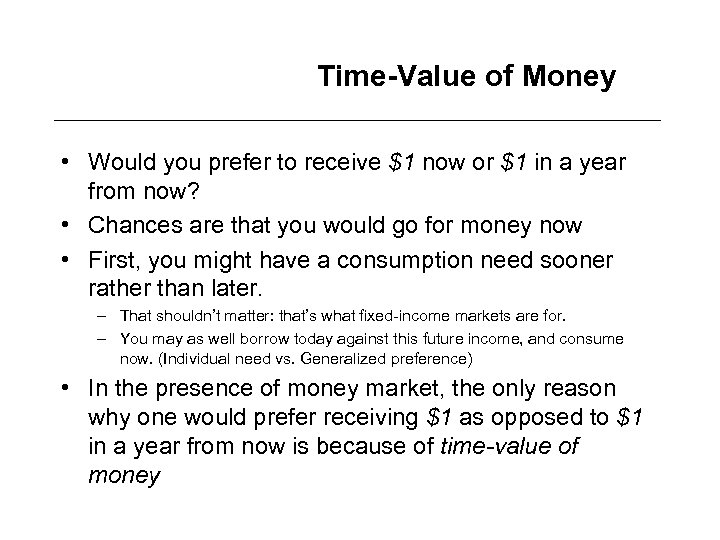Time-Value of Money • Would you prefer to receive \$1 now or \$1 in a year from now? • Chances are that you would go for money now • First, you might have a consumption need sooner rather than later. – That shouldn’t matter: that’s what fixed-income markets are for. – You may as well borrow today against this future income, and consume now. (Individual need vs. Generalized preference) • In the presence of money market, the only reason why one would prefer receiving \$1 as opposed to \$1 in a year from now is because of time-value of money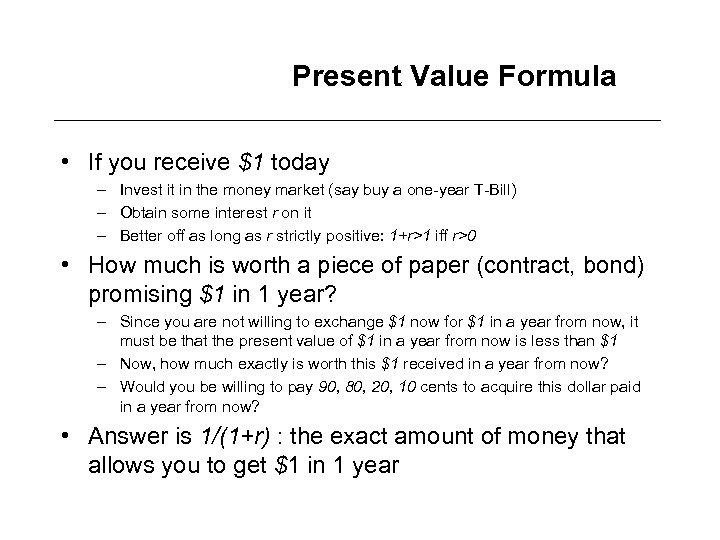Present Value Formula • If you receive \$1 today – Invest it in the money market (say buy a one-year T-Bill) – Obtain some interest r on it – Better off as long as r strictly positive: 1+r>1 iff r>0 • How much is worth a piece of paper (contract, bond) promising \$1 in 1 year? – Since you are not willing to exchange \$1 now for \$1 in a year from now, it must be that the present value of \$1 in a year from now is less than \$1 – Now, how much exactly is worth this \$1 received in a year from now? – Would you be willing to pay 90, 80, 20, 10 cents to acquire this dollar paid in a year from now? • Answer is 1/(1+r) : the exact amount of money that allows you to get \$1 in 1 year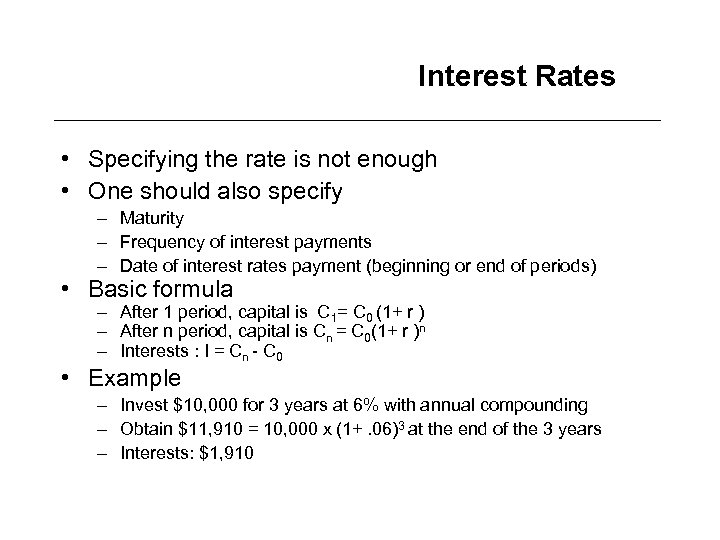Interest Rates • Specifying the rate is not enough • One should also specify – Maturity – Frequency of interest payments – Date of interest rates payment (beginning or end of periods) • Basic formula – After 1 period, capital is C 1= C 0 (1+ r ) – After n period, capital is Cn = C 0(1+ r )n – Interests : I = Cn - C 0 • Example – Invest \$10, 000 for 3 years at 6% with annual compounding – Obtain \$11, 910 = 10, 000 x (1+. 06)3 at the end of the 3 years – Interests: \$1, 910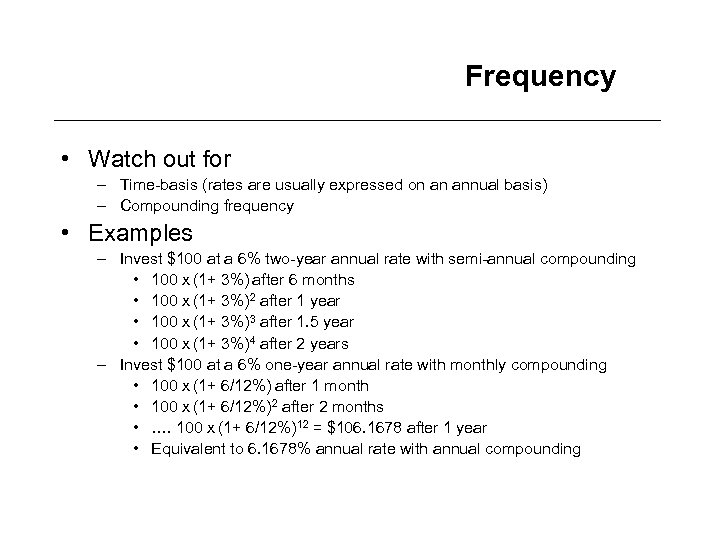Frequency • Watch out for – Time-basis (rates are usually expressed on an annual basis) – Compounding frequency • Examples – Invest \$100 at a 6% two-year annual rate with semi-annual compounding • 100 x (1+ 3%) after 6 months • 100 x (1+ 3%)2 after 1 year • 100 x (1+ 3%)3 after 1. 5 year • 100 x (1+ 3%)4 after 2 years – Invest \$100 at a 6% one-year annual rate with monthly compounding • 100 x (1+ 6/12%) after 1 month • 100 x (1+ 6/12%)2 after 2 months • …. 100 x (1+ 6/12%)12 = \$106. 1678 after 1 year • Equivalent to 6. 1678% annual rate with annual compounding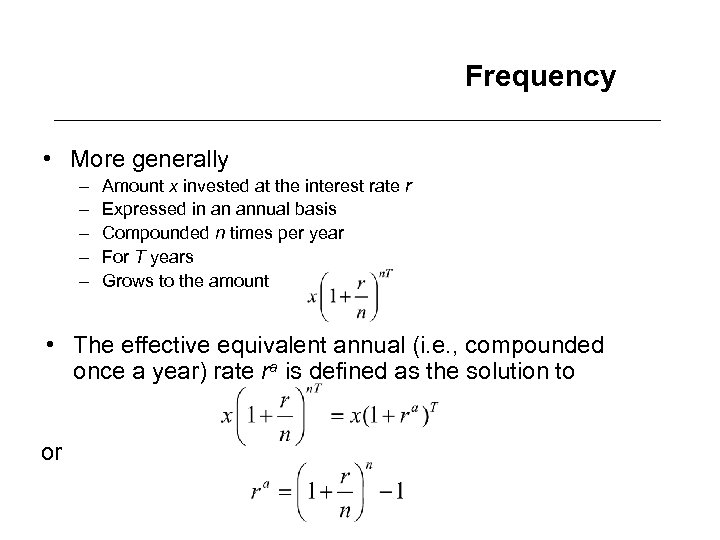Frequency • More generally – – – Amount x invested at the interest rate r Expressed in an annual basis Compounded n times per year For T years Grows to the amount • The effective equivalent annual (i. e. , compounded once a year) rate ra is defined as the solution to or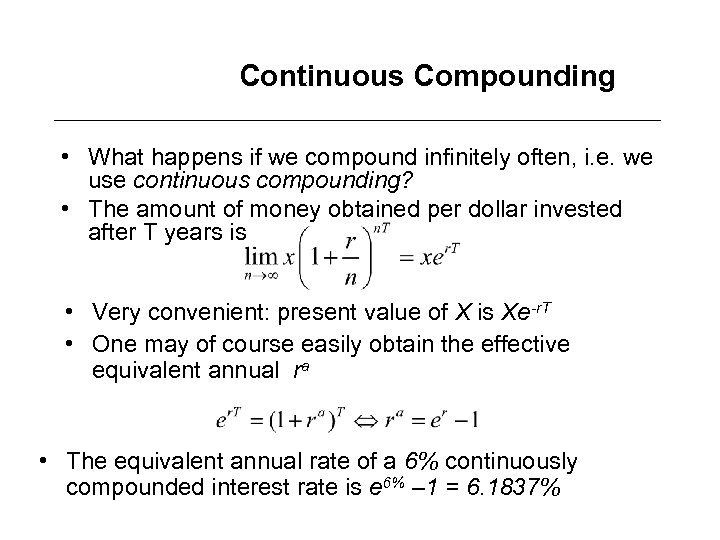Continuous Compounding • What happens if we compound infinitely often, i. e. we use continuous compounding? • The amount of money obtained per dollar invested after T years is • Very convenient: present value of X is Xe-r. T • One may of course easily obtain the effective equivalent annual ra • The equivalent annual rate of a 6% continuously compounded interest rate is e 6% – 1 = 6. 1837%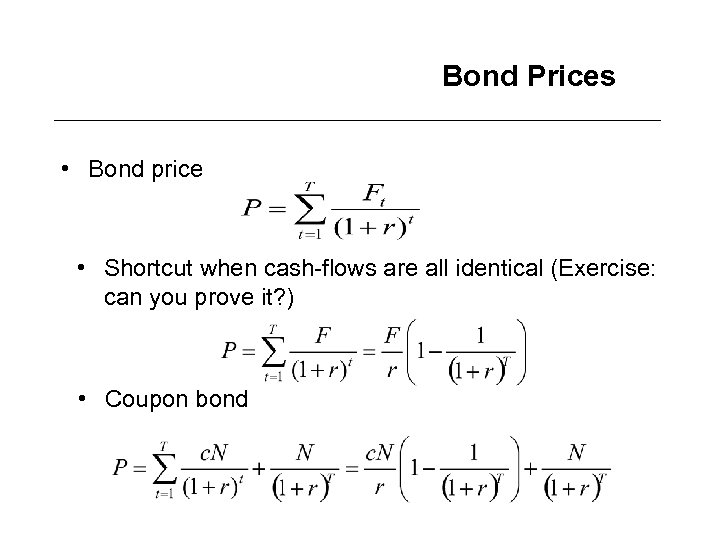Bond Prices • Bond price • Shortcut when cash-flows are all identical (Exercise: can you prove it? ) • Coupon bond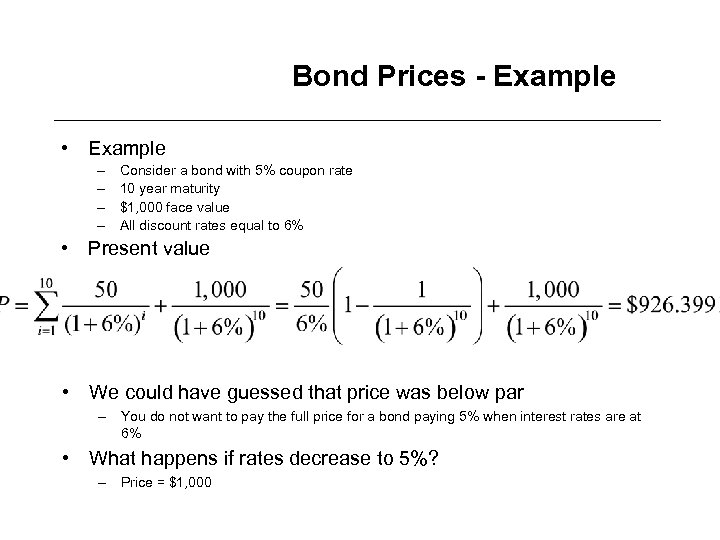Bond Prices - Example • Example – – Consider a bond with 5% coupon rate 10 year maturity \$1, 000 face value All discount rates equal to 6% • Present value • We could have guessed that price was below par – You do not want to pay the full price for a bond paying 5% when interest rates are at 6% • What happens if rates decrease to 5%? – Price = \$1, 000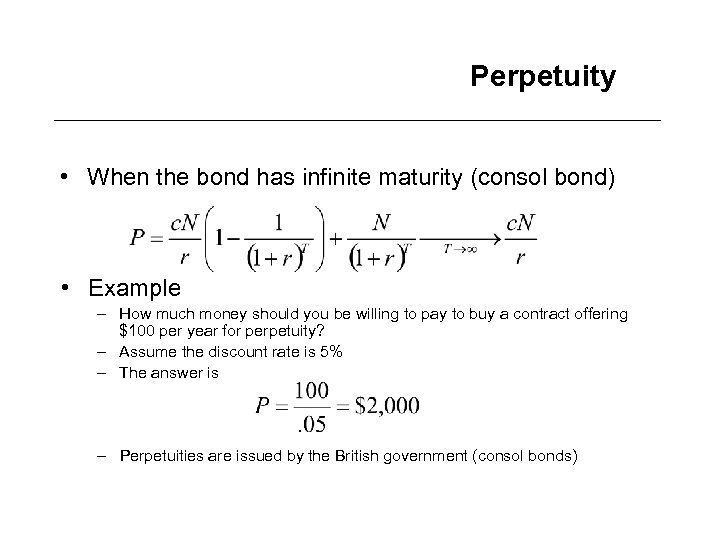Perpetuity • When the bond has infinite maturity (consol bond) • Example – How much money should you be willing to pay to buy a contract offering \$100 per year for perpetuity? – Assume the discount rate is 5% – The answer is – Perpetuities are issued by the British government (consol bonds)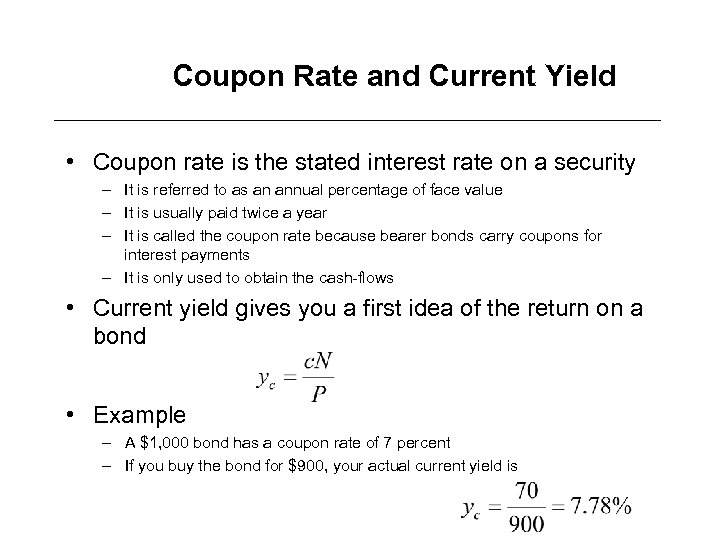Coupon Rate and Current Yield • Coupon rate is the stated interest rate on a security – It is referred to as an annual percentage of face value – It is usually paid twice a year – It is called the coupon rate because bearer bonds carry coupons for interest payments – It is only used to obtain the cash-flows • Current yield gives you a first idea of the return on a bond • Example – A \$1, 000 bond has a coupon rate of 7 percent – If you buy the bond for \$900, your actual current yield is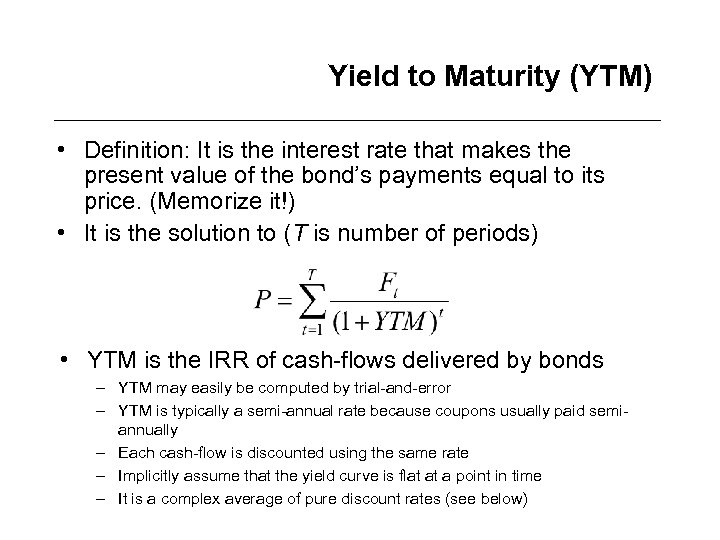Yield to Maturity (YTM) • Definition: It is the interest rate that makes the present value of the bond’s payments equal to its price. (Memorize it!) • It is the solution to (T is number of periods) • YTM is the IRR of cash-flows delivered by bonds – YTM may easily be computed by trial-and-error – YTM is typically a semi-annual rate because coupons usually paid semiannually – Each cash-flow is discounted using the same rate – Implicitly assume that the yield curve is flat at a point in time – It is a complex average of pure discount rates (see below)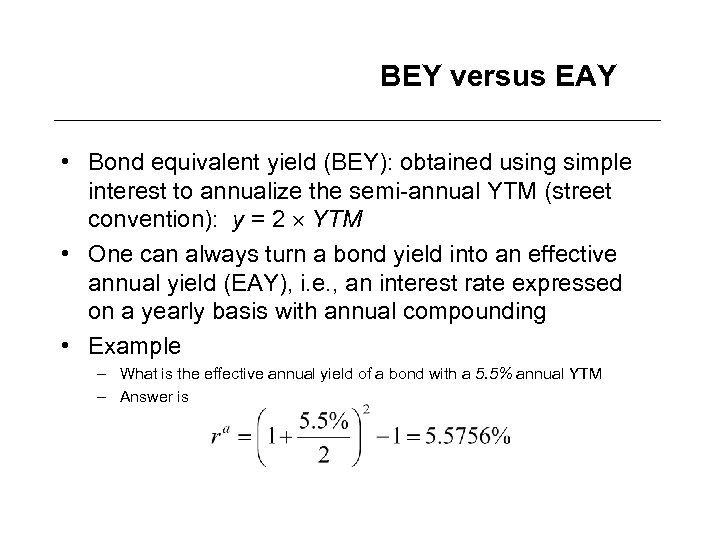BEY versus EAY • Bond equivalent yield (BEY): obtained using simple interest to annualize the semi-annual YTM (street convention): y = 2 YTM • One can always turn a bond yield into an effective annual yield (EAY), i. e. , an interest rate expressed on a yearly basis with annual compounding • Example – What is the effective annual yield of a bond with a 5. 5% annual YTM – Answer is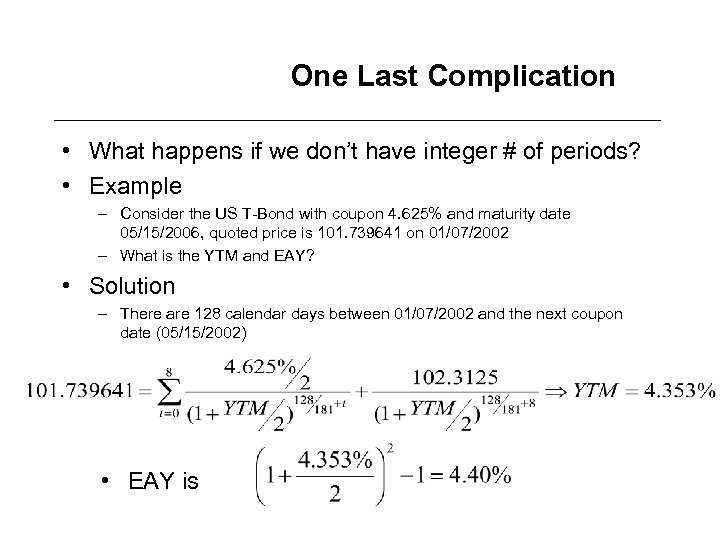One Last Complication • What happens if we don’t have integer # of periods? • Example – Consider the US T-Bond with coupon 4. 625% and maturity date 05/15/2006, quoted price is 101. 739641 on 01/07/2002 – What is the YTM and EAY? • Solution – There are 128 calendar days between 01/07/2002 and the next coupon date (05/15/2002) • EAY is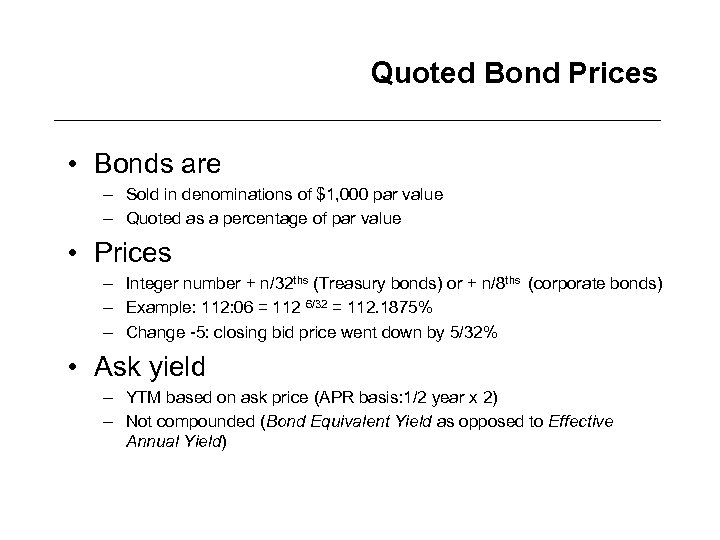Quoted Bond Prices • Bonds are – Sold in denominations of \$1, 000 par value – Quoted as a percentage of par value • Prices – Integer number + n/32 ths (Treasury bonds) or + n/8 ths (corporate bonds) – Example: 112: 06 = 112 6/32 = 112. 1875% – Change -5: closing bid price went down by 5/32% • Ask yield – YTM based on ask price (APR basis: 1/2 year x 2) – Not compounded (Bond Equivalent Yield as opposed to Effective Annual Yield)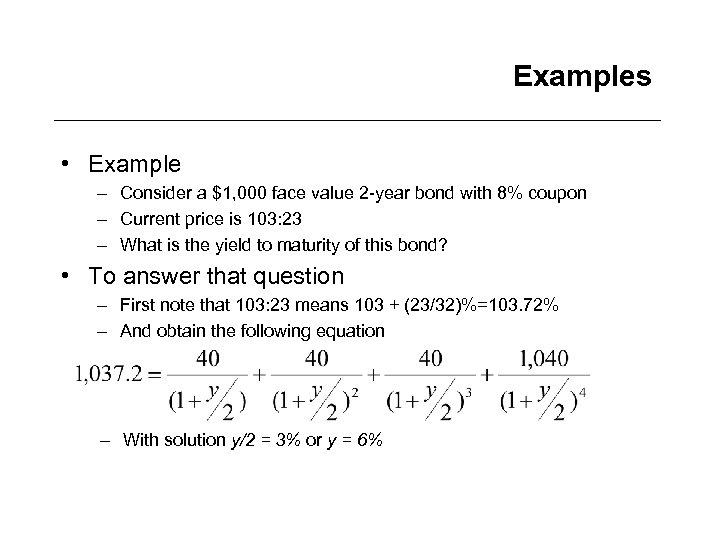Examples • Example – Consider a \$1, 000 face value 2 -year bond with 8% coupon – Current price is 103: 23 – What is the yield to maturity of this bond? • To answer that question – First note that 103: 23 means 103 + (23/32)%=103. 72% – And obtain the following equation – With solution y/2 = 3% or y = 6%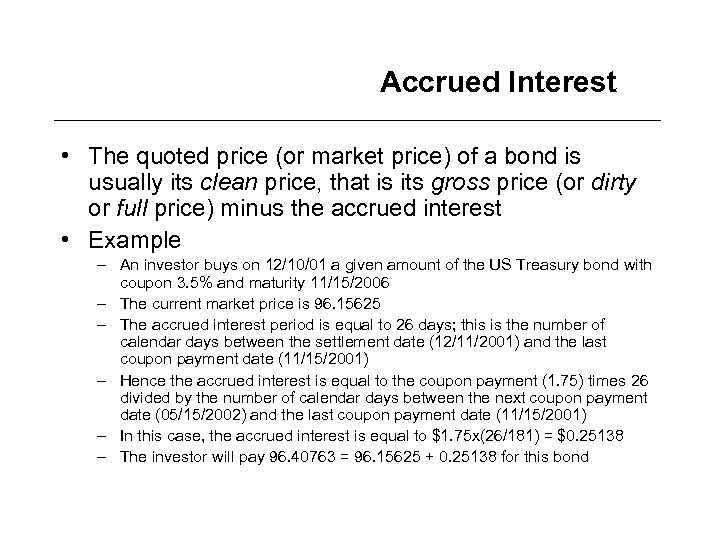Accrued Interest • The quoted price (or market price) of a bond is usually its clean price, that is its gross price (or dirty or full price) minus the accrued interest • Example – An investor buys on 12/10/01 a given amount of the US Treasury bond with coupon 3. 5% and maturity 11/15/2006 – The current market price is 96. 15625 – The accrued interest period is equal to 26 days; this is the number of calendar days between the settlement date (12/11/2001) and the last coupon payment date (11/15/2001) – Hence the accrued interest is equal to the coupon payment (1. 75) times 26 divided by the number of calendar days between the next coupon payment date (05/15/2002) and the last coupon payment date (11/15/2001) – In this case, the accrued interest is equal to \$1. 75 x(26/181) = \$0. 25138 – The investor will pay 96. 40763 = 96. 15625 + 0. 25138 for this bond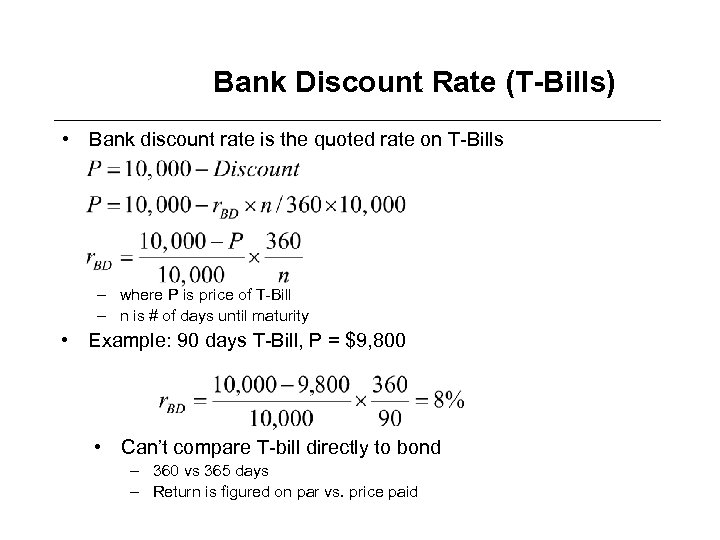Bank Discount Rate (T-Bills) • Bank discount rate is the quoted rate on T-Bills – where P is price of T-Bill – n is # of days until maturity • Example: 90 days T-Bill, P = \$9, 800 • Can’t compare T-bill directly to bond – 360 vs 365 days – Return is figured on par vs. price paid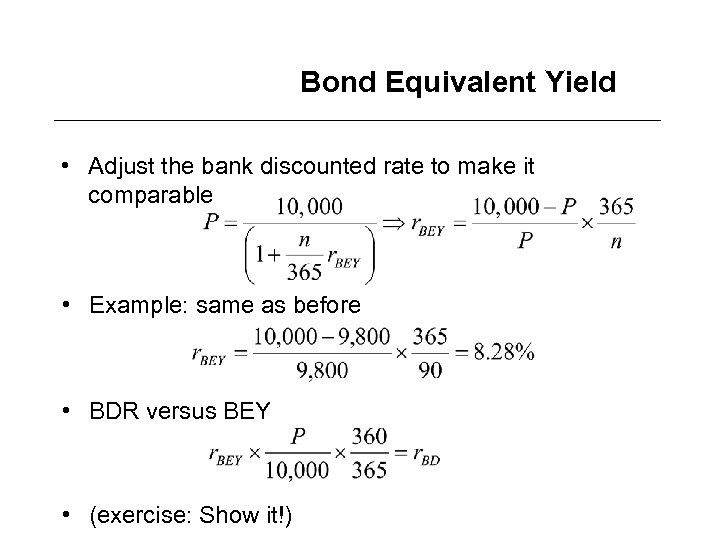Bond Equivalent Yield • Adjust the bank discounted rate to make it comparable • Example: same as before • BDR versus BEY • (exercise: Show it!)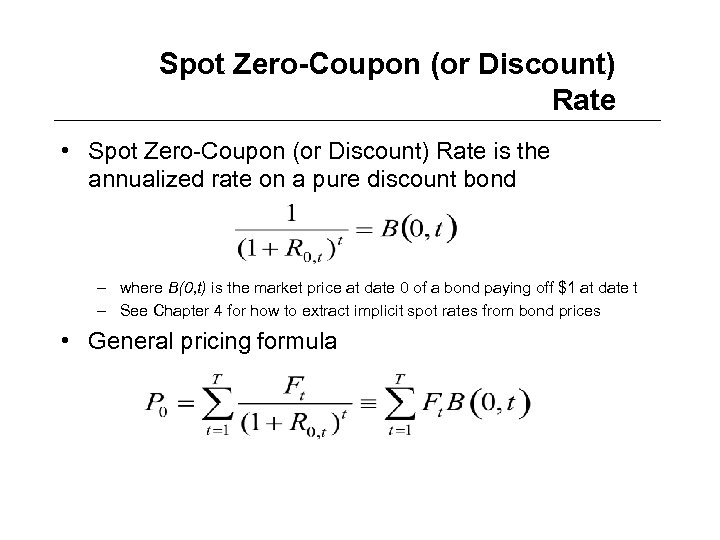Spot Zero-Coupon (or Discount) Rate • Spot Zero-Coupon (or Discount) Rate is the annualized rate on a pure discount bond – where B(0, t) is the market price at date 0 of a bond paying off \$1 at date t – See Chapter 4 for how to extract implicit spot rates from bond prices • General pricing formula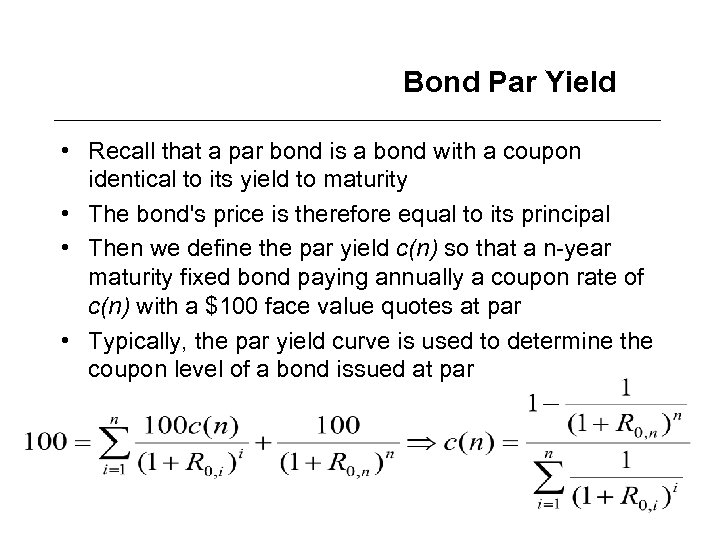Bond Par Yield • Recall that a par bond is a bond with a coupon identical to its yield to maturity • The bond's price is therefore equal to its principal • Then we define the par yield c(n) so that a n-year maturity fixed bond paying annually a coupon rate of c(n) with a \$100 face value quotes at par • Typically, the par yield curve is used to determine the coupon level of a bond issued at par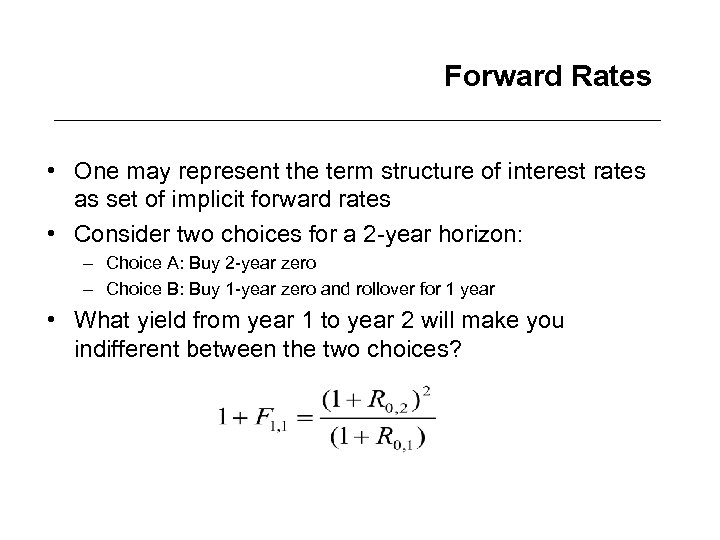Forward Rates • One may represent the term structure of interest rates as set of implicit forward rates • Consider two choices for a 2 -year horizon: – Choice A: Buy 2 -year zero – Choice B: Buy 1 -year zero and rollover for 1 year • What yield from year 1 to year 2 will make you indifferent between the two choices?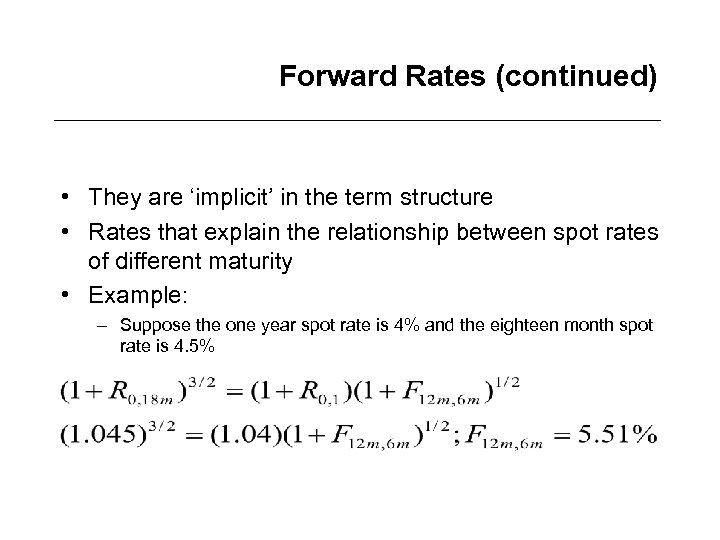Forward Rates (continued) • They are ‘implicit’ in the term structure • Rates that explain the relationship between spot rates of different maturity • Example: – Suppose the one year spot rate is 4% and the eighteen month spot rate is 4. 5%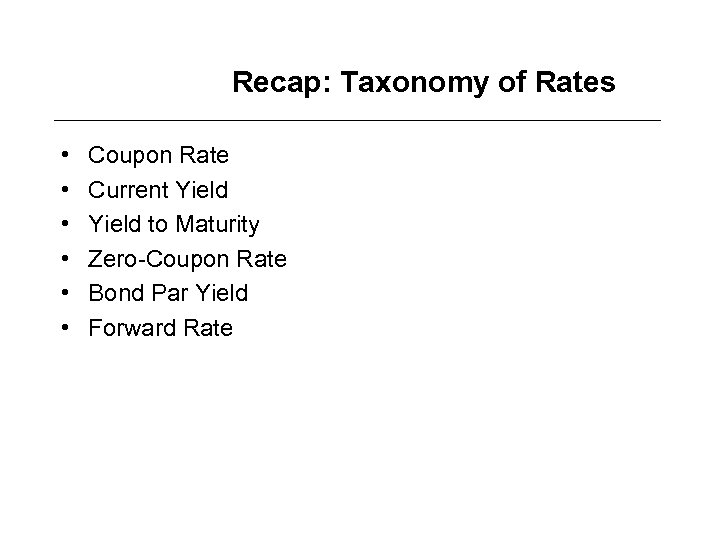Recap: Taxonomy of Rates • • • Coupon Rate Current Yield to Maturity Zero-Coupon Rate Bond Par Yield Forward Rate